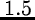Next: MODEL/ROMAFOT Up: How to use ROMAFOT Previous: CHECK/ROMAFOT

Big Pixels

This problem is examined in Buonanno and Iannicola, 1989. In short, ROMAFOT performs the best fit by comparing a given pixel to the value of the PSF at the centre of the pixel. This approximation is valid if the pixel size is small compared with the scale length of the point images. If R is the ratio between the FWHM of the PSF and the pixel size, then a value ofis the limit of validity of the quoted approximation and, correspondingly, the limit beyond which we enter in the regime of big pixels. With the commands below the integral of the PSF over the pixel area is computed via the Gauss - Legendre integration formulae which are characterized by a high precision for a correspondingly small number of subpixels where the PSF must be calculated.

Since the number of subpixels depends on the intensity of the star, on the local gradient of the PSF and so on, two commands are used. The first command prepares a file where the subpixel values are computed according to the different parameters of the problem (aperture of the telescope, exposure time, seeing, required accuracy, magnitude of the star, distance of the pixel from the centre of the star and so forth). The second command performs the non - linear fitting by comparing the integral of the PSF over the subpixel values.Next: MODEL/ROMAFOT Up: How to use ROMAFOT Previous: CHECK/ROMAFOT
Petra Nass
1999-06-15